SCPS198C September   2014  – February 2017

PRODUCTION DATA.

1. Features
2. Applications
3. Description
4. Revision History
5. Pin Configuration and Functions
6. Specifications
7. Parameter Measurement Information
8. Detailed Description
1. 8.1 Overview
2. 8.2 Functional Block Diagram
3. 8.3 Feature Description
4. 8.4 Device Functional Modes
5. 8.5 Programming
6. 8.6 Register Maps
2. 8.6.2 Control Register and Command Byte
3. 8.6.3 Register Descriptions
1. 8.6.3.1 Bus Transactions
9. Application and Implementation
1. 9.1 Application Information
2. 9.2 Typical Application
10. 10Power Supply Recommendations
11. 11Layout
12. 12Device and Documentation Support
13. 13Mechanical, Packaging, and Orderable Information

• DW|16
• PW|16
• DW|16

## 9 Application and Implementation

NOTE

Information in the following applications sections is not part of the TI component specification, and TI does not warrant its accuracy or completeness. TI’s customers are responsible for determining suitability of components for their purposes. Customers should validate and test their design implementation to confirm system functionality.

### 9.1 Application Information

Figure 33 shows an application in which the TCA9534A can be used.

IO Expanders such as the TCA9534A are commonly used to obtain more general purpose I/Os. There are many common uses for these additionial I/Os:

• Inputs from other ICs, such as interrupt signals from sensors
• Inputs from physical buttons (for detecting button presses)
• Outputs to control RESET or ENABLE signals on other ICs
• Outputs for controlling LEDs for visual feedback to a user

### 9.2 Typical Application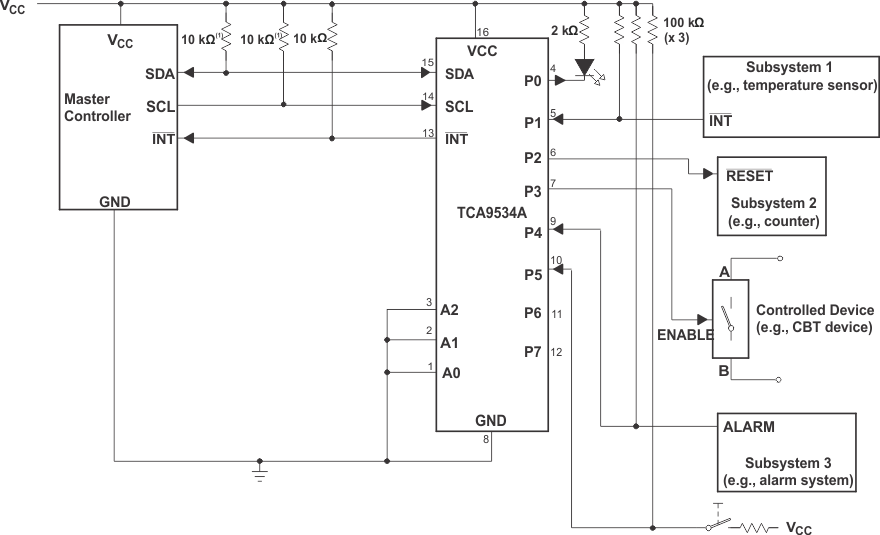The SCL and SDA pins must be tied directly to VCC because if SCL and SDA are tied to an auxiliary power supply that can be powered on while VCC is powered off, then the supply current, ICC, increases as a result.
Device address is configured as 0111000 for this example.
P0, P2, and P3 are configured as outputs.
P1, P4, and P5 are configured as inputs.
P6 and P7 are not used and must be configured as outputs.
Figure 33. Application Schematic

#### 9.2.1.1 Calculating Junction Temperature and Power Dissipation

When designing with the TCA9534A, it is important that the Recommended Operating Conditions not be violated. Many of the parameters of this device are rated based on junction temperature. So junction temperature must be calculated in order to verify that safe operation of the device is met. The basic equation for junction temperature is shown in Equation 1.

Equation 1.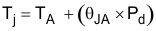θJA is the standard junction to ambient thermal resistance measurement of the package, as seen in Thermal Information table. Pd is the total power dissipation of the device, and the approximation is shown in Equation 2.

Equation 2.Equation 2 is the approximation of power dissipation in the device. The equation is the static power plus the summation of power dissipated by each port (with a different equation based on if the port is outputting high, or outputting low. If the port is set as an input, then power dissipation is the input leakage of the pin multiplied by the voltage on the pin). Note that this ignores power dissipation in the INT and SDA pins, assuming these transients to be small. They can easily be included in the power dissipation calculation by using Equation 3 to calculate the power dissipation in INT or SDA while they are pulling low, and this gives maximum power dissipation.

Equation 3.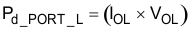Equation 3 shows the power dissipation for a single port which is set to output low. The power dissipated by the port is the VOL of the port multiplied by the current it is sinking.

Equation 4.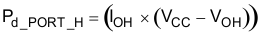Equation 4 shows the power dissipation for a single port which is set to output high. The power dissipated by the port is the current sourced by the port multiplied by the voltage drop across the device (difference between VCC and the output voltage).

#### 9.2.1.2 Minimizing ICC When I/Os Control LEDs

When the I/Os are used to control LEDs, normally they are connected to VCC through a resistor as shown in Figure 33. For a P-port configured as an input, ICC increases as VI becomes lower than VCC. The LED is a diode, with threshold voltage VT, and when a P-port is configured as an input the LED is off but VI is a VT drop below VCC.

For battery-powered applications, it is essential that the voltage of P-ports controlling LEDs is greater than or equal to VCC when the P-ports are configured as input to minimize current consumption. Figure 34 shows a high-value resistor in parallel with the LED. Figure 35 shows VCC less than the LED supply voltage by at least VT. Both of these methods maintain the I/O VI at or above VCC and prevents additional supply current consumption when the P-port is configured as an input and the LED is off.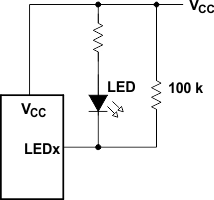Figure 34. High-Value Resistor in Parallel With LED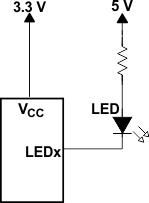Figure 35. Device Supplied by a Lower Voltage

#### 9.2.2 Detailed Design Procedure

The pull-up resistors, RP, for the SCL and SDA lines need to be selected appropriately and take into consideration the total capacitance of all slaves on the I2C bus. The minimum pull-up resistance is a function of VCC, VOL,(max), and IOL as shown in Equation 5.

Equation 5.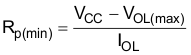The maximum pull-up resistance is a function of the maximum rise time, tr (300 ns for fast-mode operation, fSCL = 400 kHz) and bus capacitance, Cb as shown in Equation 6.

Equation 6.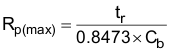The maximum bus capacitance for an I2C bus must not exceed 400 pF for standard-mode or fast-mode operation. The bus capacitance can be approximated by adding the capacitance of the TCA9534A, Ci for SCL or Cio for SDA, the capacitance of wires, connections, traces, and the capacitance of additional slaves on the bus.

#### 9.2.3 Application Curves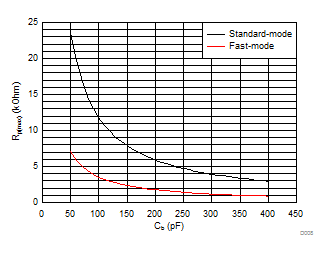Standard-mode (fSCL = 100 kHz, tr = 1 µs) Fast-mode (fSCL = 400 kHz, tr = 300 ns)
Figure 36. Maximum Pull-Up resistance (Rp(max)) vs Bus Capacitance (Cb)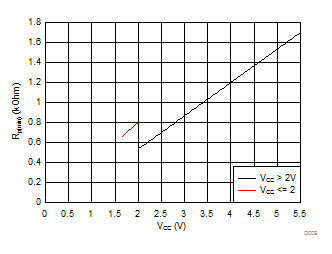VOL = 0.2*VCC, IOL = 2 mA when VCC ≤ 2 V VOL = 0.4 V, IOL = 3 mA when VCC > 2 V
Figure 37. Minimum Pull-Up Resistance (Rp(min)) vs Pull-Up Reference Voltage (VCC)